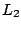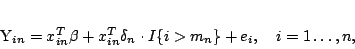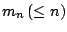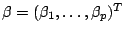# Use of Permutation Principle in detection of structural changes in regression

Jaromír Antoch

Charles University of Prague, Department of Statistics, Sokolovská 83,
CZ-18675 Praha 8-Karlín, Czech Republic
jaromir.antoch@karlin.mff.cuni.cz

Keywords: Linear regression, structural changes,-procedures, permutation principle, Monte Carlo, change-point problem.

We consider the regression model with a change after an unknown time point, i.e.(1)

where,andare unknown parameters,are known design points andare iid random errors fulfilling regularity conditions specified below. Functiondenotes the indicator of the set.

Model () describes the situation where the firstobservations follow the linear model with the parameterand the remainingobservations follow the linear regression model with the parameter. The parameteris usually called the change point.

In the lecture we focus on the testing problem:(2)

Approximations to the critical values needed for this testing problem can be obtained through the limit distribution of the respective test statistics under, however, such approximations are usually not satisfactory. Therefore, we proposed another possibility, namely, the approximations based on the application of the permutational principle, of course, suitably modified for the situation of regression models. We will discuss this approach in the lecture and give some examples based on real data illustrating its advantages and disadvantages.

2005-05-23